## Riemann Hypothesis

First published in Riemann (1859), the Riemann hypothesis states that the nontrivial Roots of the Riemann Zeta Function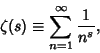(1)

where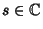(the Complex Numbers), all lie on the Critical Line''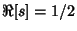, where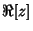denotes the Real Part of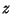. The Riemann hypothesis is also known as Artin's Conjecture.

In 1914, Hardyproved that an Infinite number of values for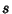can be found for which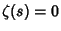and. However, it is not known if all nontrivial rootssatisfy, so the conjecture remains open. André Weil proved the Riemann hypothesis to be true for field functions (Weil 1948, Eichler 1966, Ball and Coxeter 1987). In 1974, Levin showed that at least 1/3 of the Roots must lie on the Critical Line (Le Lionnais 1983), a result which has since been sharpened to 40% (Vardi 1991, p. 142). It is known that the zeros are symmetrical placed about the line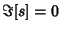.

The Riemann hypothesis is equivalent to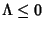, where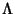is the de Bruijn-Newman Constant (Csordas et al. 1994). It is also equivalent to the assertion that for some constant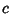,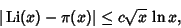(2)

where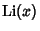is the Logarithmic Integral and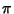is the Prime Counting Function (Wagon 1991).

The hypothesis was computationally tested and found to be true for the first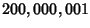zeros by Brent et al. (1982). Brent's calculation covered zeros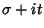in the region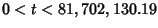.

There is also a finite analog of the Riemann hypothesis concerning the location of zeros for function fields defined by equations such as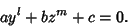(3)

This hypothesis, developed by Weil, is analogous to the usual Riemann hypothesis. The number of solutions for the particular cases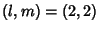, (3,3), (4,4), and (2,4) were known to Gauß.See also Critical Line, Extended Riemann Hypothesis, Gronwall's Theorem, Mertens Conjecture, Mills' Constant, Riemann Zeta Function

References

Ball, W. W. R. and Coxeter, H. S. M. Mathematical Recreations and Essays, 13th ed. New York: Dover, p. 75, 1987.

Brent, R. P. On the Zeros of the Riemann Zeta Function in the Critical Strip.'' Math. Comput. 33, 1361-1372, 1979.

Brent, R. P.; van de Lune, J.; te Riele, H. J. J.; and Winter, D. T. On the Zeros of the Riemann Zeta Function in the Critical Strip. II.'' Math. Comput. 39, 681-688, 1982. Abstract available at ftp://nimbus.anu.edu.au/pub/Brent/rpb070a.dvi.Z.

Csordas, G.; Smith, W.; and Varga, R. S. Lehmer Pairs of Zeros, the de Bruijn-Newman Constant and the Riemann Hypothesis.'' Constr. Approx. 10, 107-129, 1994.

Eichler, M. Introduction to the Theory of Algebraic Numbers and Functions. New York: Academic Press, 1966.

Le Lionnais, F. Les nombres remarquables. Paris: Hermann, p. 25, 1983.

Odlyzko, A. The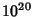th Zero of the Riemann Zeta Function and 70 Million of Its Neighbors.''

Riemann, B. Über die Anzahl der Primzahlen unter einer gegebenen Grösse,'' Mon. Not. Berlin Akad., pp. 671-680, Nov. 1859.

Sloane, N. J. A. Sequence A002410/M4924 in An On-Line Version of the Encyclopedia of Integer Sequences.'' http://www.research.att.com/~njas/sequences/eisonline.html and Sloane, N. J. A. and Plouffe, S. The Encyclopedia of Integer Sequences. San Diego: Academic Press, 1995.

van de Lune, J. and te Riele, H. J. J. On The Zeros of the Riemann Zeta-Function in the Critical Strip. III.'' Math. Comput. 41, 759-767, 1983.

van de Lune, J.; te Riele, H. J. J.; and Winter, D. T. On the Zeros of the Riemann Zeta Function in the Critical Strip. IV.'' Math. Comput. 46, 667-681, 1986.

Wagon, S. Mathematica in Action. New York: W. H. Freeman, p. 33, 1991.

Weil, A. Sur les courbes algébriques et les variétès qui s'en déduisent. Paris, 1948.